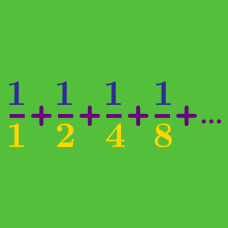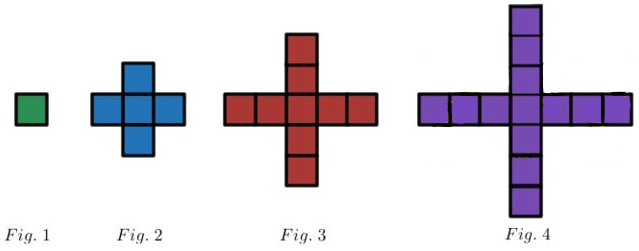Calculus

# Sequences and Series: Level 1 ChallengesHow many squares are present in figure number 20?

When I was a teenager, a friend and I stole soda from our parents. I took the soda bottle, drank half of what's there, and then passed it to my friend. My friend drank half of what was left, and passed it back to me. I again drank half of what was left, and passed it back to him.
We kept up with this until the entire soda bottle was empty.

Approximately what percentage of the soda did I drink?

$\frac{100001+100003+100005+\cdots+199999}{1+3+5+7+\cdots+99999} = \, ?$

$\left(\dfrac1{2^2}+\dfrac1{3^2}+\dfrac1{4^2}+\ldots\right) + \\ \hspace{1cm}\left(\dfrac1{2^3}+\dfrac1{3^3}+\dfrac1{4^3} + \ldots\right) + \\ \hspace{2.5cm}\left(\dfrac1{2^4}+\dfrac1{3^4}+\dfrac1{4^4}+\ldots\right)+\cdots$

What is the value of the series above?

A girl puts $1$ grain of rice in the first square of an 8 by 8 chess board. In the subsequent square, she puts twice that of the previous square, and she continues until she fills all the squares.

How many total grains does she need?×